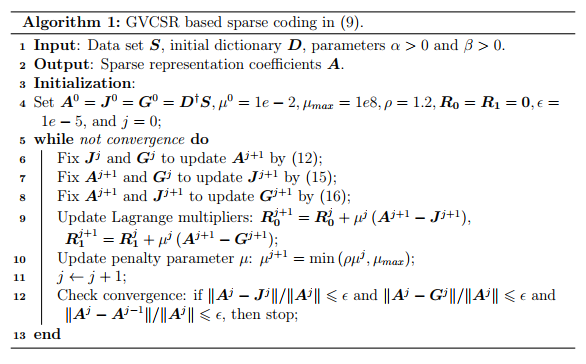# Globally Variance-Constrained Sparse Representation for Rate-Distortion Optimized Image Representation### Abstract

Sparse representation is efficient to approximately recover signals by a linear composition of a few bases from an over-complete dictionary. However, in the scenario of data compression, its efficiency and popularity are hindered due to the extra overhead for encoding the sparse coefficients. Therefore, how to establish an accurate rate model in sparse coding and dictionary learning becomes meaningful, which has been not fully exploited in the context of sparse representation. According to the Shannon entropy inequality, the variance of data source can bound its entropy, thus can reflect the actual coding bits. Therefore, a Globally Variance-Constrained Sparse Representation (GVCSR) model is proposed, where a variance-constrained rate term is introduced to the conventional sparse representation. To solve the non-convex optimization problem, we employ the Alternating Direction Method of Multipliers (ADMM) for sparse coding and dictionary learning, both of which have shown state-of-the-art rate-distortion performance in image representation.

Type
Publication
Data Compression Conference